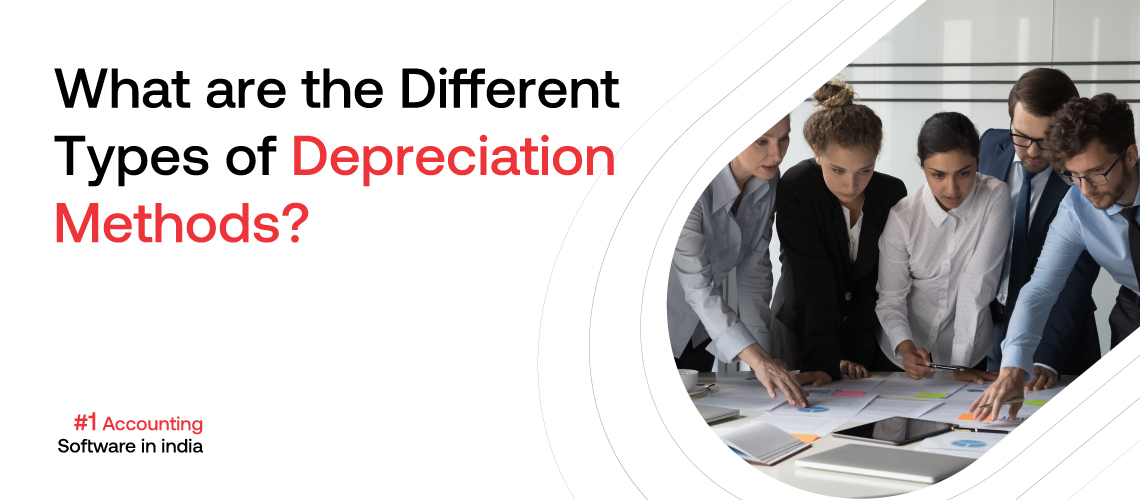# What are the Different Types of Depreciation Methods?Depreciation is a term that business owners hear all the time, but what does it mean? Depreciation is the process of allocating the cost of an asset over its useful life. This allows you to write off a portion of the asset's cost each year, saving you money on your taxes.

There are a few different types of depreciation methods, and each has its benefits and drawbacks. In this blog, we'll understand each type of depreciation and know how you can leverage accounting software for small business to calculate the depreciation on your assets.

## What is Depreciation?

Depreciation is a word used in accounting to describe the periodic reduction of a fixed asset's recorded cost until the asset's value is zero or insignificant.

When you purchase an asset for your business—whether it's a piece of machinery or real estate—you'll want to account for the fact that it will lose value over time. This is what depreciation is all about.

## Methods of Depreciation

There are two main methods of depreciation: straight line and double declining balance.

Straight-line depreciation is the simplest method, where you divide the asset's cost by its useful life. For example, if you purchase a machine for ₹1,00,000 and its estimated useful life is five years, you would calculate depreciation as ₹20,000 per year. This is just an example, and you would need to consult with an accountant or take the help of a small business accounting software.

Double declining balance depreciation is more accelerated, where you double the depreciation in the early years and then decline it gradually as the asset gets closer to the end of its life.

## Straight Line Depreciation

Straight-line depreciation is the simplest of the two, and it's also the most common. Under this method, the value of an asset is reduced by an equal amount each year. So, if you buy a computer for ₹1,000, your annual deduction would be ₹100.

There are a few advantages to using straight-line depreciation. First, it's easy to calculate. Secondly, it provides a consistent deduction each year, which can be helpful for budgeting purposes.

However, there are also a few drawbacks. One is that it doesn't reflect the true value of an asset. This means you could be overpaying taxes in the early years and missing out on deductions later.

## Declining Balance Depreciation

Under this method, the depreciation rate is based on the balance of the asset's original cost that's still outstanding. So, for example, if your asset is worth ₹1,000 and you've already written off ₹600 of that cost, your depreciation rate for the next year will be based on the remaining ₹400 balance.

The key advantage of this method is that it allows you to write off a larger portion of the asset's cost in the early years when it's likely to have the most impact on your business. Another advantage is that it's relatively easy to calculate. You multiply the depreciation rate by the original cost of the asset.

One downside of this method is that it doesn't consider the asset's current market value. So, you could write off more than the asset is worth.

Another potential downside is that because the depreciation rate is based on the balance of the original cost, it can fluctuate from year to year.

### Conclusion

When it comes to depreciation methods, there are two main types: straight-line depreciation and declining balance depreciation. Each method has its benefits and drawbacks, so it's important to understand the pros and cons of each before choosing one for your business.

Harness the power of robust accounting software for small businessthat can help you calculate depreciation automatically and accurately.